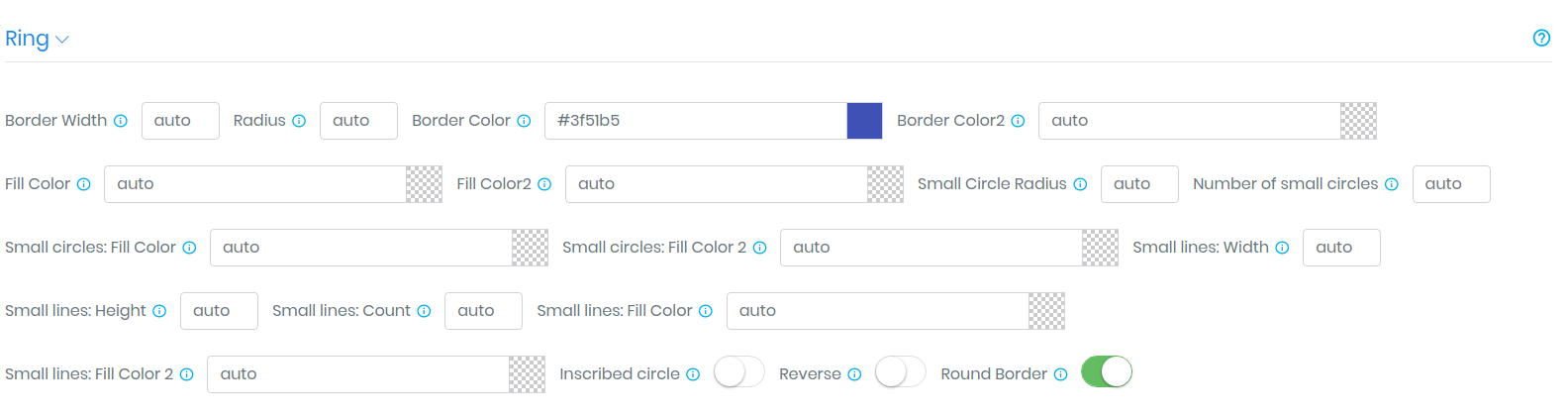Tutorial

# Custom Design: Ring

The "Ring" block is used to customize the design of the circles describing the counter segments around. It consists of such controllers:

1. "Border Width" - the size of the frame of a circle.
3. "Border Color" - the color of the upper border of the circle.
4. "Border Color2" - the color of the lower border of the circle.
5. "Fill Color" - fill the circle.
6. "Fill Color 2" - color changing area.
7. "Small Circle Radius" - the radius of the small circles describing the date segment.
8. "Number of small circles" - number of small circles The number of small circles describing the date segment.
9. "Small circles: Fill Color" - primary color of the small circle.
10. "Small circles: Fill Color 2" - color replacing the main, time-dependent.
11. "Small lines: Width" - the width of the lines described in a circle date segment.
12. "Small lines: Height" - the height of the lines described in a circle date segment.
13. "Small lines: Count" - the number of lines described in the circular segment of the date.
14. "Small lines: Fill Color" - the main color of the lines described in the round date segment.
15. "Small lines: Fill Color 2" - color replacing the main, time-dependent..
16. "Padding" - rectangle indents, with the help of them you can set additional gaps between adjacent objects of the counter.
17. "Inscribed circle" - fits a circle into a segment, mainly used if you want to make a smaller radius of your circle relative to the counter segment.
18. "Reverse" - changes the course of a circle frame animation.
19. "Round Border" - Rounds a circle border.

Remark - for resizing the radius of a circle, use the "Padding" field in the "Segment Rectangle" block